# Google Code Jam Archive — Round 1A 2008 problems

## Overview

The first sub-round of Google Code Jam 2008 Round 1 featured three little challenges. Each problem required one or two cute ideas to solve.

The first problem is formulated in one of the important concepts connecting algebra and geometry, that of scalar product, also known as inner product. Though the problem itself is an exercise in arrays and numbers.

The second problem, as mundane as the name suggests, has some connection to the propositional logic, especially the satisfiability problem. Unlike the super-hard general satisfiability problem, this one is in a restricted form, and thus allows one to find a simple and cute solution.

The third problem, appears as a numerical problem, actually should be solved with only integer numbers. With ideas from elementary algebra, combinatorics, and number theory, armed with some standard tricks on the computational side, we know at least four different solutions with distinct flavor.

2394 contestants finished the match with a positive score, among them, we have 41 perfect scores.

Credits

Problem A. Minimum Scalar Product Written by Michael Levin. Prepared by Frank Chu.

Problem B. Milkshakes Written by John Dethridge. Prepared by Frank Chu and John Dethridge.

Problem C. Numbers Written and prepared by Cosmin Negruseri.

Contest analysis presented by Cosmin Negruseri, Xiaomin Chen, and John Dethridge.

## A. Minimum Scalar Product

### Problem

You are given two vectors v1 = (x1, x2, ..., xn) and v2 = (y1, y2, ..., yn). The scalar product of these vectors is a single number, calculated as x1y1 + x2y2 + ... + xnyn.

Suppose you are allowed to permute the coordinates of each vector as you wish. Choose two permutations such that the scalar product of your two new vectors is the smallest possible, and output that minimum scalar product.

### Input

The first line of the input file contains integer number T - the number of test cases. For each test case, the first line contains integer number n. The next two lines contain n integers each, giving the coordinates of v1 and v2 respectively.

### Output

For each test case, output a line

`Case #X: Y`
where X is the test case number, starting from 1, and Y is the minimum scalar product of all permutations of the two given vectors.

### Limits

Time limit: 30 seconds per test set.
Memory limit: 1GB.

#### Small dataset (Test set 1 - Visible)

T = 1000
1 ≤ n ≤ 8
-1000 ≤ xi, yi ≤ 1000

#### Large dataset (Test set 2 - Hidden)

T = 10
100 ≤ n ≤ 800
-100000 ≤ xi, yi ≤ 100000

### Sample

Sample Input
```2
3
1 3 -5
-2 4 1
5
1 2 3 4 5
1 0 1 0 1
```
Sample Output
```Case #1: -25
Case #2: 6
```

## B. Milkshakes

### Problem

You own a milkshake shop. There are N different flavors that you can prepare, and each flavor can be prepared "malted" or "unmalted". So, you can make 2N different types of milkshakes.

Each of your customers has a set of milkshake types that they like, and they will be satisfied if you have at least one of those types prepared. At most one of the types a customer likes will be a "malted" flavor.

You want to make N batches of milkshakes, so that:

• There is exactly one batch for each flavor of milkshake, and it is either malted or unmalted.
• For each customer, you make at least one milkshake type that they like.
• The minimum possible number of batches are malted.

Find whether it is possible to satisfy all your customers given these constraints, and if it is, what milkshake types you should make.

If it is possible to satisfy all your customers, there will be only one answer which minimizes the number of malted batches.

### Input

One line containing an integer C, the number of test cases in the input file.
For each test case, there will be:

• One line containing the integer N, the number of milkshake flavors.
• One line containing the integer M, the number of customers.
• M lines, one for each customer, each containing:
• An integer T ≥ 1, the number of milkshake types the customer likes, followed by
• T pairs of integers "X Y", one for each type the customer likes, where X is the milkshake flavor between 1 and N inclusive, and Y is either 0 to indicate unmalted, or 1 to indicated malted. Note that:
• No pair will occur more than once for a single customer.
• Each customer will have at least one flavor that they like (T ≥ 1).
• Each customer will like at most one malted flavor. (At most one pair for each customer has Y = 1).
All of these numbers are separated by single spaces.

### Output

C lines, one for each test case in the order they occur in the input file, each containing the string "Case #X: " where X is the number of the test case, starting from 1, followed by:

• The string "IMPOSSIBLE", if the customers' preferences cannot be satisfied; OR
• N space-separated integers, one for each flavor from 1 to N, which are 0 if the corresponding flavor should be prepared unmalted, and 1 if it should be malted.

### Limits

Time limit: 30 seconds per test set.
Memory limit: 1GB.

C = 100
1 ≤ N ≤ 10
1 ≤ M ≤ 100

#### Large dataset (Test set 2 - Hidden)

C = 5
1 ≤ N ≤ 2000
1 ≤ M ≤ 2000

The sum of all the T values for the customers in a test case will not exceed 3000.

### Sample

Sample Input
```2
5
3
1 1 1
2 1 0 2 0
1 5 0
1
2
1 1 0
1 1 1
```
Sample Output
```Case #1: 1 0 0 0 0
Case #2: IMPOSSIBLE
```

In the first case, you must make flavor #1 malted, to satisfy the first customer. Every other flavor can be unmalted. The second customer is satisfied by getting flavor #2 unmalted, and the third customer is satisfied by getting flavor #5 unmalted.

In the second case, there is only one flavor. One of your customers wants it malted and one wants it unmalted. You cannot satisfy them both.

## C. Numbers

### Problem

In this problem, you have to find the last three digits before the decimal point for the number (3 + √5)n.

For example, when n = 5, (3 + √5)5 = 3935.73982... The answer is 935.

For n = 2, (3 + √5)2 = 27.4164079... The answer is 027.

### Input

The first line of input gives the number of cases, T. T test cases follow, each on a separate line. Each test case contains one positive integer n.

### Output

For each input case, you should output:

`Case #X: Y`
where X is the number of the test case and Y is the last three integer digits of the number (3 + √5)n. In case that number has fewer than three integer digits, add leading zeros so that your output contains exactly three digits.

### Limits

Time limit: 30 seconds per test set.
Memory limit: 1GB.
1 ≤ T ≤ 100

2 ≤ n ≤ 30

#### Large dataset (Test set 2 - Hidden)

2 ≤ n ≤ 2000000000

### Sample

Sample Input
```2
5
2
```
Sample Output
```Case #1: 935
Case #2: 027
```

## Analysis — A. Minimum Scalar Product

In spite of the geometric flavor in the name of this problem, it is truly a story of two arrays.

There are two permutations involved. However, after you fix the permutation for V1, you have complete freedom in choosing the permutation for V2. It is clear that the first permutation really does not matter. The important thing is which xi gets to be matched to which yj.

To make thinking easier, we may assume that the first permutation has

```x1 ≤ x2 ≤ ... ≤ xn. (1)
```

And our task is to match the y's to the x's so that the scalar product is as small as possible.

At this point, if you need a little exercise in the middle: Think about the case n = 2, and just use a concrete example. What you will surely discover is that, in order to achieve the minimum scalar product, you always want to match the smaller xi with the bigger yj.

What we would like to prove is: under condition (1), one of the optimal solutions is of the form

```  y1 ≥ y2 ≥ ... ≥ yn. (2)
```

The rigorous proof of (2) is given at the end of this analysis. For an easy reading, we point out that the key step is really the n = 2 case. If x < x' and y < y', then

```(xy + x'y') - (xy' + x'y) = (x - x')(y - y') > 0. (*)
```

So we prefer to match bigger y's with smaller x's.

Therefore, this problem is solved by the following simple algorithm.

```sort(v1.begin(), v1.end());
sort(v2.begin(), v2.end(), greater<int>());
long long ret = 0;
for (int i = 0; i < n; i++)
ret += (long long)(v1[i]) * v2[i];
```

Proof of (2). We prove that any permutation that does not satisfy (2) can be transformed into one satisfying (2), and in each step we do not increase the scalar product.
Indeed, any unsorted array can be transformed to a sorted one by only interchanging adjacent elements that are out of order. (For more rigorous readers: prove this, maybe by counting the number of flipped pairs in the array.)
At each step, some x = xi is matched to some y, and x' = xi+1 is matched to some y' so that y ≤ y'. We interchange y and y' in this step. The inequality similar to (*), with > replaced by ≥, tells us that the scalar product is not increased in this step. ◊

Test Data
info We recommend that you practice debugging solutions without looking at the test data.

## Analysis — B. Milkshakes

On the surface, this problem appears to require solving the classic problem "Satisfiability," the canonical example of an NP-complete problem. The customers represent clauses, the milkshake flavors represent variables, and malted and unmalted flavors represent whether the variable is negated.

We are not evil enough to have chosen a problem that hard! The restriction that makes this problem easier is that the customers can only like at most one malted flavor (or equivalently, the clauses can only have at most one negated variable.)

Using the following steps, we can quickly find whether a solution exists, and if so, what the solution is.

1. Start with every flavor unmalted and consider the customers one by one.
2. If there is an unsatisfied customer who only likes unmalted flavors, and all those flavors have been made malted, then no solution is possible.
3. If there is an unsatisfied customer who has one favorite malted flavor, then we must make that flavor malted. We do this, then go back to step 2.
4. If there are no unsatisfied customers, then we already have a valid solution and can leave the remaining flavors unmalted.

Notice that whenever we made a flavor malted, we were forced to do so. Therefore, the solution we got must have the minimum possible number of malted flavors.

With clever data structures, the above algorithm can be implemented to run in linear time.

Test Data
info We recommend that you practice debugging solutions without looking at the test data.

## Analysis — C. Numbers

This problem with a simple statement was in fact one of the hardest in Round 1. The input restrictions for the small input were chosen so that straightforward solutions in Java or Python, which have arbitrary-precision numbers, would fail. The trick was calculating √5 to a large enough precision and not using the default double value. It turned out that using the Windows calculator or the UNIX `bc` tool was enough to solve the small tests as well.

Solving the large tests was a very different problem. The difficulty comes from the fact that √5 is irrational and for n close to 2000000000 you would need a lot of precision and a lot of time if you wanted to use the naive solution.

The key in solving the problem is a mathematical concept called conjugation. In our problem, we simply note that (3 - √5) is a nice conjugate for (3 + √5). Let us define

(1)     α := 3 + √5,   β := 3 - √5,   and Xn := αn + βn.

We first note that Xn is an integer. This can be proved by using the binomial expansion. If you write everything down you'll notice that the irrational terms of the sums cancel each other out.

(2)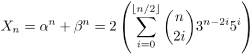Another observation is that βn < 1, so Xn is actually the first integer greater than αn. Thus we may just focus on computing the last three digits of X.

A side note. In fact, βn tends to 0 so quickly that that our problem would be trivial if we asked for the three digits after the decimal point. For all large values of n they are always 999.

Based on (1) and (2), there are many different solutions for finding the last three digits of Xn.

### Solution A. [the interleave of rational and irrational]

One solution goes like this: αn can be written as (an + bn√5), where an and bn are integers. At the same time, βn is exactly (an - bn√5) and Xn = 2an. Observe that

(3)     α(n + 1) = (3 + √5)(an + bn√5) = (3an + 5bn) + (3bn + an)√5.

So an + 1 = 3an + 5bn and bn + 1 = 3bn + an. This can be written in matrix form as

(4)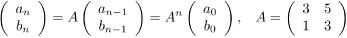Since α0 = 1, we have (a0, b0) = (1, 0).

Now we use the standard fast exponentiation to get An in O(log n) time. Note that we do all operations modulo 1000 because we just need to return the last three digits of an.

Here's some Python code that implements this solution:

```def matrix_mult(A, B):
C = [[0, 0], [0, 0]]
for i in range(2):
for j in range(2):
for k in range(2):
C[i][k] = (C[i][k] + A[i][j] * B[j][k]) % 1000
return C

def fast_exponentiation(A, n):
if n == 1:
return A
else:
if n % 2 == 0:
A1 = fast_exponentiation(A, n/2)
return matrix_mult(A1, A1)
else:
return matrix_mult(A, fast_exponentiation(A, n - 1))

def solve(n):
A = [[3, 5], [1, 3]]
A_n = fast_exponentiation(A, n)
return (2 * M_n + 999) % 1000
```

### Solution B. [the quadratic equation and linear recurrence]

Experienced contestants may notice there is a linear recurrence on the Xi's. Indeed, this is not hard to find -- the conjugation enters the picture again.

Notice that

(5)     α + β = 6, and α β = 4.

So α and β are the two roots of the quadratic equation x2 - 6x + 4 = 0. i.e.,

(6)     α2 = 6α - 4, and β2 = 6β - 4.

Looking at (1) and (6) together, we happily get

(7)     Xn+2 = 6Xn+1 - 4Xn.

Such recurrence can always be written in matrix form. It is somewhat redundant, but it is useful: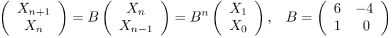From here it is another fast matrix exponentiation. Let us see radeye's perl code that implements this approach here:

```sub mul {
my \$a = shift ;
my \$b = shift ;
my @a = @{\$a} ;
my @b = @{\$b} ;
my @c = (\$a*\$b + \$a*\$b,
\$a*\$b + \$a*\$b,
\$a*\$b + \$a*\$b,
\$a*\$b + \$a*\$b) ;
@c = map { \$_ % 1000 } @c ;
return @c ;
}
sub f {
my \$n = shift ;
return 2 if \$n == 0 ;
return 6 if \$n == 1 ;
return 28 if \$n == 2 ;
\$n -= 2 ;
my @mat = (0, 1, 996, 6) ;
my @smat = @mat ;
while (\$n > 0) {
if (\$n & 1) {
@mat = mul([@mat], [@smat]) ;
}
@smat = mul([@smat], [@smat]) ;
\$n >>= 1 ;
}
return (\$mat * 6 + \$mat * 28) % 1000 ;
}
sub ff {
my \$r = shift ;
\$r = (\$r + 999) % 1000 ;
\$r = "0" . \$r while length(\$r) < 3 ;
return \$r ;
}
for \$c (1..<>) {
\$n = <> ;
print "Case #\$c: ", ff(f(\$n)), "\n" ;
}
```

### Solution C. [the periodicity of 3 digits]

For this problem, we have another approach based on the recurrence (7). Notice that we only need to focus on the last 3 digits of Xn, which only depends on the last 3 digits of the previous two terms. The numbers eventually become periodic as soon as we have (Xi, Xi+1) and (Xj, Xj+1) with the same last 3 digits, where i < j. It is clear that we will enter a cycle no later than 106 steps. In fact, for this problem, you can write some code and find out that the cycle has the size 100 and starts at the 3rd element in the sequence. So to solve the problem we can just brute force the results for the first 103 numbers and if n is bigger than 103 return the result computed for the number (n - 3) % 100 + 3.

### Solution D. [the pure quest of numbers and combinatorics]

Let us see one more solution of different flavor. Here is a solution not as general as the others, but tailored to this problem, and makes one feel we are almost solving this problem by hand.

Let us look again at (2). We want to know Xn mod 1000. We know from the chinese remander theorem that if we can find Xn mod 8 and Xn mod 125, then Xn mod 1000 is uniquely determined.
(a) For n > 2, Xn mod 8 is always 0. Since 5i ≡ 1 (mod 4), 3n-2i ≡ 1 or -1 (mod 4) depending on n, so, for n > 2,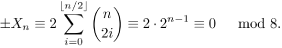(b) To compute Xn mod 125, we only need to worry about i=0,1,2. All the rest are 0 mod 125. In other words, all we need to compute is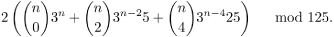There are various ways to compute the elements in the above quantity. The exponents can be computed by fast exponentiation, or using the fact that 3n mod 125 is periodic with cycle length at most 124. The binomial numbers can be computed using arbitrary precision integers in languages like Java and Python, or with a bit careful programming in languages like C++.

Test Data
info We recommend that you practice debugging solutions without looking at the test data.

## Statistics — A. Minimum Scalar Product

### Test set 1: 2352 correct solutions (98.2% solve rate)

First
yuhch123 C++, 4:22
RalphFurmaniak C++, 5:00
mtakeshi 5:35
bee_ aka bee C++, 5:45
ASotelo Java, 5:58
Shortest
rsotolongo Pascal, 60 bytes
code0012 C++, 80 bytes
uru Ruby, 195 bytes
linguo Perl, 198 bytes

### Test set 2: 1048 correct solutions (43.8% solve rate)

First
yuhch123 C++, 4:22
bee_ aka bee C++, 5:45
ASotelo Java, 5:58
Bewildered turquoise ferret 5:59
Martial 6:05
Shortest
code0012 C++, 80 bytes
uru Ruby, 195 bytes
pancho -, 248 bytes
irori Ruby, 253 bytes

## Statistics — B. Milkshakes

### Test set 1: 659 correct solutions (27.5% solve rate)

First
Olexiy C++, 21:34
jdmetz C++, 22:08
yuhch123 C++, 22:48
bhzhan aka Bohua C++, 24:07
connect4 C++, 24:22
Shortest
yanghuaisheng C++, 404 bytes
morefreeze aka MoreFreeze C++, 507 bytes
irori Ruby, 699 bytes
wjsw C++, 887 bytes
domeng C++, 888 bytes

### Test set 2: 312 correct solutions (13.0% solve rate)

First
Olexiy C++, 21:34
yuhch123 C++, 22:48
bhzhan aka Bohua C++, 24:07
tgm C++, 24:43
pashka Java, 25:33
Shortest
irori Ruby, 699 bytes
Omoikane C++, 940 bytes
battyone C++, 999 bytes
bug C++, 1014 bytes
jasonw Lisp, 1035 bytes

## Statistics — C. Numbers

### Test set 1: 577 correct solutions (24.1% solve rate)

First
youresam Java, 12:29
linguo Plsql, 17:00
Eddie Python, 19:29
Amused lime ifrit 21:01
KOTEHOK Java, 21:07
Shortest
RoBa -, 108 bytes
Piluex -, 145 bytes
crem Perl, 167 bytes
talchas Bash, 211 bytes
KirarinSnow -, 229 bytes

### Test set 2: 96 correct solutions (4.0% solve rate)

First
Plagapong C++, 31:13
fuwenjie C++, 31:46
Graceful lavender jackal 33:21